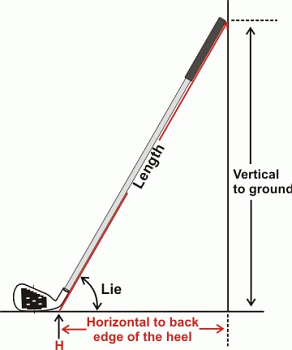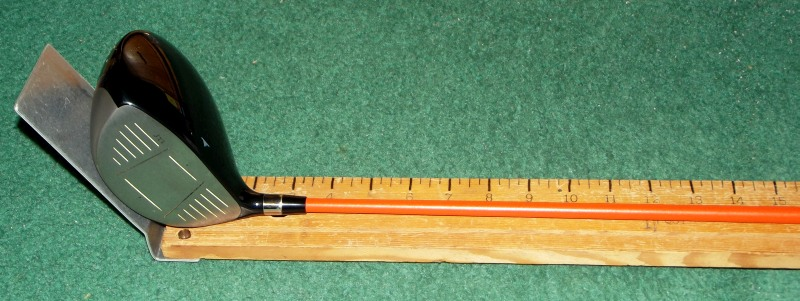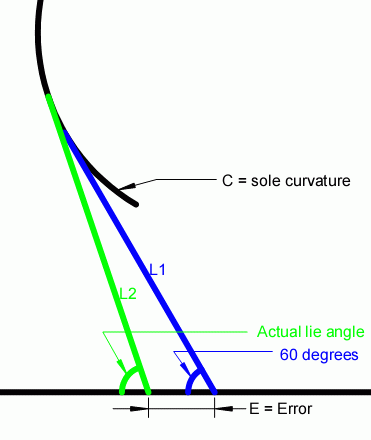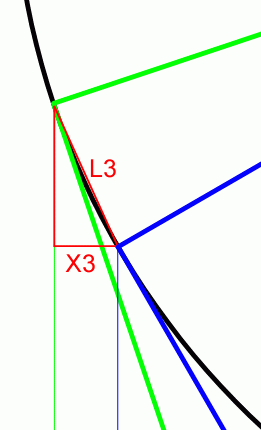# Club Ruler - New vs Old

Dave Tutelman -- December 6, 2011

As of 2004, the USGA and R&A introduced a rule specifying how the length of a golf club is to be measured. They did this in order to be able to impose a maximum length to a club. When it comes right down to it, they wanted to limit the length of drivers, as part of their effort to control the distance that the best players hit the ball.

This had an interesting effect on the design of shop equipment, specifically the club ruler. There was already in place a traditional method for measuring club length, and every clubmaking shop had a club ruler designed to implement the traditional method. With the new method called for in the Rules of Golf, manufacturers of clubmaking instruments put new club rulers in their catalogs, designed to implement the USGA/R&A rule.

The question arises: "How different are the measurements of the old ruler and the new ruler?" This article answers that question.

The short form of the answer is: generally less than 1/12" (2mm). For more information than that, you'll have to read the article.

## The Old WayLet's start with the old method of measurement. Here is a picture from an article by Jeff Summitt at the Hireko web site showing the traditional way. The length was measured along the shaft, from the floor to the butt of the grip, with the head level. That meant that the angle of the shaft (and the angle of the ruler) was the lie angle of the club.

Instrument makers built their club rulers to this method. The example shown below is from GolfMechanix, and is typical of a pre-2004 club ruler. The shaft of the club lies along the ruler, and the sole of the clubhead presses against a hinged stop. The angle of the stop is adjusted so that it equals the lie angle. With the sole resting against a stop set at the lie angle, the geometry is exactly the same as the diagram to the left. The length measured along the ruler is the length of the club.## The New Way

Now along come the rule-makers, who want to put a limit on the maximum length of a driver. Being experienced rule-makers, they don't want any loopholes, any way to "game" the rules. And one way to game the rules is to lie about the lie -- that is, to assure that the lie angle as measured is very different from the lie angle as played. This is easy to do for a driver. Consider:
• Lie measurement is usually taken assuming that the scorelines on the face are perfectly horizontal at address. And that is the case, if the clubhead was made without any ulterior motives. But...
• Grooves do nothing for a driver. Launch conditions are not influenced at all by a smooth face, horizontal grooves, vertical grooves, or grooves at any angle you want.
So the way to "game" the length measurement is to put seriously slanted scorelines on the face. If lie angle is measured (as it usually is) with scorelines level, you can make the measurement occur at a very flat lie angle, with no compulsion to actually play the club that way. The result is that the club measures maybe an inch shorter than it plays -- and it beats the length limit by an inch.

In order to head off such gaming, the rule makers said the angle of the sole stop had to be 60º -- as opposed to hinged and matched to the lie angle. 60º is well within the range of lie angles across the bag, and closer to the driver end of the bag. Sounds like a reasonable way to measure, if the goal is to control maximum driver length.So a lot of clubmakers and instrument makers said, "OK, there's a new way to measure club length. Let's redesign the club ruler to this new method." Certainly worthwhile if you need to be sure you are building a legal driver. Here is a picture of GolfMechanix' product to meet that need. It has a non-hinged solid sole stop, cut at a 60º angle. This actually has a number of advantages over the old way:
• Inexpensive. Certainly less expensive than a good hinged stop.
• Easy to use. No need to adjust the stop to align it with the scorelines, in order to get the lie angle right.
• Harder to misuse. You can't accidentally bump the stop to a flatter angle as you are setting the club in the ruler. (Don't laugh; that has been done, and not infrequently.)
But you will notice that the photos show an iron being measured, not a driver. So it is being used as the primary club ruler, not just a check on legality. When you use it for your club ruler, you should want to know if it gives you the same reading as the traditional club ruler.

## What's the Difference?No, really! What is the difference between the length measured by an old ruler and a new one? Here is a graph of the difference for typical clubs. The assumptions made in producing the graph include:
• Drivers have lie angles between 56º and 60º, with the majority at 58º. (The graph shows the whole range, but we should probably use 58º for most purposes.)
• The highest lie in the set is 64º, the specification for most wedges.
• The sole curvature is about 4 degrees per inch. (Below, we test sensitivity to this assumption, over the range of 3 to 6 degrees per inch.)
• The distance along the stop where the sole hits is 1.7", when the stop is set to the lie angle. (I measured a bunch of clubs, and they were between 1.5" and 1.8". 1.7" is toward the upper end of the range. Below, we test sensitivity to this assumption.)
• The graph assumes the traditional method gives the correct answer, and we want to find the difference due to the new method. If we want to view it the other way around, just change the sign of the difference.
(The equations to draw this graph come from the analysis in the Appendix. The equations were implemented in Excel to produce the graph, and to run the sensitivity analysis below.)

The immediate conclusion to be drawn from the graph is that the error is less than 1/8" (.125"), and usually much less. If we limit the lie to the usual driver lie of 58º, the error is barely above 1/16" (.063"). A sixteenth of an inch is tighter than I control club length in my shop, so it is plenty good enough for me. I suspect that is true for the vast majority of shops.

(Side note: I know clubmakers who are completely anal-retentive about getting every measurement to the last decimal place. The usual justification, when you dig down, is "because I can". Feel free to do your thing, guys. But now you have a decision to make: which method is the right measurement of length. I can't help you there.)

### "I'm a pioneer"

In the late 1990s, I did a back-of-the-envelope calculation that told me the error due to a fixed stop could be limited to about 1/8". It was not nearly as careful an analysis as this one, but I knew it was not too far off. I could see the advantages as being easy to make and maintain, easy to use, and not very error-prone.Based on that, I built the club ruler shown in the photo for my own shop, with a stop of sheet aluminum bent to 60º. I have been using it to measure club length ever since.

### Sensitivity analysis

Our conclusion is that, over the lie range from 58º to 64º, the difference between the two rulers is less than .075" -- just over 1/16" and just under 2mm -- maximum. But there are two assumptions that may be driving the answer, so we should see how the answer changes when we change the assumptions. The assumptions we are going to test are:
• Curvature of the sole: we will test using 3 to 6 degrees per inch.
• Effective length of the stop: we will test using 1.5 to 1.9 inches.
 Differences: Sensitivity to assumptions Curvature (degrees per inch) Length of stop (inches) Driver (lie = 58º) Wedge (lie = 64º) 1.5 1.7 1.9 1.5 1.7 1.9 3 .055 .061 .067 -.052 -.064 -.076 4 .052 .058 .064 -.062 -.074 -.086 5 .051 .057 .063 -.068 -.080 -.093 6 .050 .056 .062 -.072 -.084 -.097
The results are in the table at right. The table shows the extremes of the curve, the driver at 58º and the wedge at 64º. The difference are smaller for all the other clubs. Of course, the difference is always zero at 60º lie; the stops are at identical angles.

Observations:
• In every case, the difference was less than 0.1", a tenth of an inch.
• The differences are larger for larger stop lengths and more curvature. In my measuring, I found that high-curvature clubs tended to have smaller stop lengths, so the high end of the differences would not have been encountered with real clubs. Therefore a more realistic estimate of the maximum difference you would encounter is about 0.08", about a twelfth of an inch.

## Conclusion

The length measured by the new rule is not very different from the old. The difference in every realistic case for normal clubheads is less than 0.08" (or 2.0mm). The new method is a simpler and more foolproof operation, lower cost, and with greater potential to build it yourself. And it comes at very little accuracy penalty, if the old method is considered "correct".

### Appendix: Analysis of the difference

(Feel free to skip this if you aren't interested in where the numerical results came from.)Here is the geometry of the problem we are trying to solve. The elements are:
• The ruler body, represented by the black horizontal line.
• The sole of the club, represented by the black arc.
• The 60º stop is a blue line tangent to the sole, at a 60º angle (naturally) to the ruler body.
• The lie-angle stop is a green line tangent to the sole. Its angle to the ruler body is equal to the lie angle.
Each of these elements has some measure associated with it:
• The sole of the club has a curvature, denoted as C. The curvature of the sole is expressed in degrees per inch; that is, how many degrees does the direction change for each inch moved from heel to toe along the sole? Most clubfitters are familiar with bending lie angle based on scuff marks on the sole; the usual rule of thumb is one degree per quarter inch off center. That implies a curvature of one degree per quarter inch, or C=4. Much to my surprise, the number was fairly similar with the drivers I measured. So we'll use C=4, and check the sensitivity to C when we're done.
• The distance along the stop, from the ruler to contact with the sole, is L1 for the 60º stop and L2 for the true lie angle. I measured a bunch of clubs and found L2 to be in the range of 1.5" to 1.8".
• What we want to find is the difference, or error, between the two measurements. That is the distance along the ruler from the green line to the blue line, shown as E on the diagram.Let's look at the problem in more detail. We'll complete the green and blue right triangles, and see what we can deduce. Let's label distances along the ruler (or even just parallel to the ruler) as X distances. So:
• The blue distance, corresponding to L1, is X1.
• The green distance, corresponding to L2, is X2.
• The horizontal component of the distance between the tangent points is X3, shown in red. (We'll identify another right triangle later, that will enable us to compute X3.)
A quick inspection of this diagram gives us an equation for the error:

 E + X2 = X1 + X3

or

 E = X1 - X2 + X3

The rest of this analysis consists of finding the values of X1, X2, and X3 so we can calculate E.

Another observation about the diagram: the distance between the blue and green tangent points subtends an angle along the arc which we will call d. A few interesting points from that observation:
• d is equal to the difference between 60º and the actual lie angle.
• The distance along the arc can be computed, since we know the curvature in degrees per inch. That distance is d/C.
For purposes of the work that follows, let's keep the blue angle more general than 60º. We'll call it a. We can always set a=60º when we need a numerical value.

We will call the lie angle b, and remember that b=a+d.

On to finding the Xs...

From basic trigonometry:

X1 = L1 cos(a)

X2 = L2 cos(b)

The difference between L1 and L2 is very close to the length of the arc between the tangents. We already identified the arc length as equal to d/C and angle b as a+d. So we can closely approximate:

L1 = L2 - d/C

Therefore:

X1 = (L2 - d/C) cos(a)

X2 = L2 cos(a+d)All we need now is X3. To find it, we will take a closer look at the area where the stops are tangent to the sole curve. We can draw another right triangle, this one shown in red. If we knew L3 and  the angle between X3 and L3, we could compute X3 easily. So let's try to find L3 and the angle.

First L3. It is the chord between the tangents. Since the arc covers very few degrees, the chord and the arc are almost the same length, which we know to be d/C.

Now, what do we know about that angle? Just from inspection, it must be larger than a and smaller than b. It looks to be about halfway between them. Actually, it is not hard to prove that it is exactly halfway between a and b, which is (a+d/2).

Therefore:

 X3 = d C cos(a+d/2)

We now have expressions for X1, X2, and X3 that we can plug into the expression for E.

 E =  X1 - X2 + X3 = (L2 - d/C) cos(a)  -  L2 cos(a+d)  +  (d/C) cos(a+d/2)

A word about the sign (direction) of the error. As it stands, this equation treats measurement at 60º as the correct way, and measurement with a stop at the lie angle to be in error. But we came at this with a traditionalist view: "What sort of error does the new club ruler introduce, assuming we were measuring things correctly before." So, when we plot the graphs, we will plot -E, which is the error introduced by the new ruler.